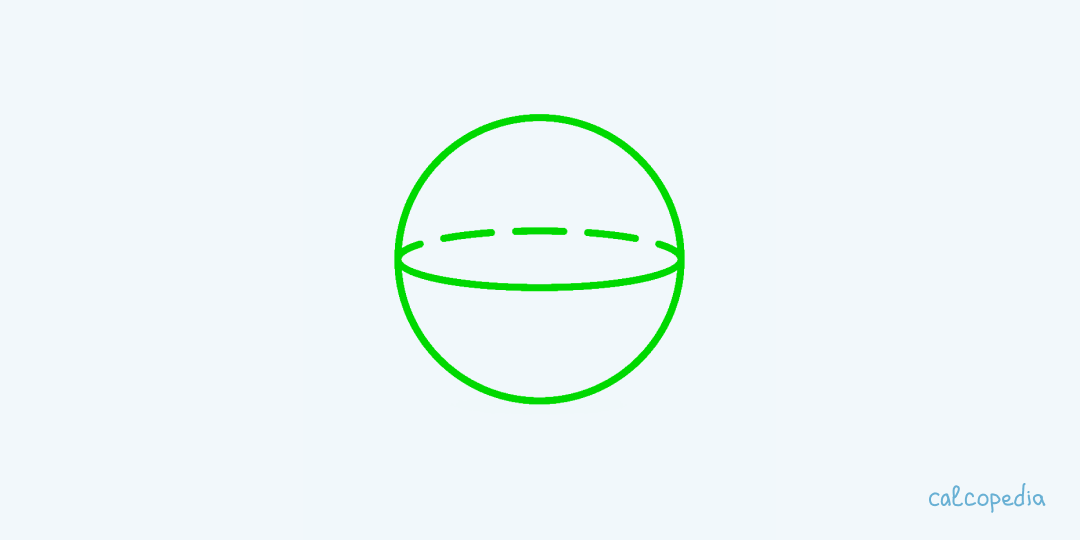# Sphere Surface Area Calculator

Calculate the surface area of a spherical object (sphere) using our online calculator.

Sphere Surface Area:

0copy

Contents

## What is the Surface Area of a Sphere and How to Calculate It?The surface area of a sphere refers to the total area that covers its exterior. Imagine if you could peel an orange and lay its skin flat – the area of this skin represents the surface area of the sphere.

The formula to calculate the surface area of a sphere is 4πr², where r represents the radius of the sphere.

The concept of the sphere`s surface area is fundamental in various fields, including physics, engineering, and even arts. Knowing how to determine this area is essential for tasks ranging from painting a round object to calculating the heat transfer across a spherical boundary.

With advancements in technology, we no longer have to rely solely on manual calculations. Online calculators, like the one we're introducing here, make these computations quick and easy.

## How to Use the Sphere Surface Area Calculator

Our online calculator is designed to be user-friendly, but if you're new to this, here`s a step-by-step guide to get you started:

1. Open the calculator interface.

2. You will see an input box labeled 'Radius'. Enter the value of the radius of the sphere.

3. Ensure that you've selected the correct unit of measurement (e.g., meters, centimeters).

4. Click on the 'Calculate' button.

5. The calculator will immediately provide you with the surface area, calculated using the formula mentioned above.

6. If you wish, you can reset the values and start over.

7. Always double-check the entered values for accuracy before using the results for practical applications.

## Examples of Calculating the Surface Area of a Sphere

Learning by example can sometimes be the most effective way. Let`s delve into some practical scenarios:

Example 1: The Playful Balloon - Let`s say you've bought a perfectly round balloon with a radius of 7 cm for a kid`s birthday party. Using our formula, 4π(7²) = 615.75 cm². So, the total surface area of the balloon is approximately 615.75 cm². That`s a lot of area for those funny clown faces!

Example 2: The Giant Chocolate Ball - Imagine a giant chocolate ball with a radius of 10 cm (a chocoholic`s dream!). The surface area? 4π(10²) = 1,256.64 cm². That`s quite the canvas for intricate chocolate designs!

Example 3: Planet Earth for Dummies - Well, Earth isn`t a perfect sphere, but for the sake of simplicity, let`s say it has an average radius of 6,371 km. The surface area becomes a whopping 510 million km²! No wonder it takes so long to travel the world.

## Nuances in Calculating the Surface Area of a Sphere

While our calculator makes things easy, there are some nuances you should be aware of:

1. Always ensure the radius value is accurate. A slight error can result in significant discrepancies in the calculated area.
2. The units matter! Converting between cm, m, km, and other units can affect the result. Always use consistent units.
3. Real-world objects might not be perfect spheres. The formula assumes a perfectly round shape.
4. External factors like pressure and temperature can slightly change the shape (and hence the surface area) of real-world spherical objects.
5. For rough surfaces, the "visible" surface area might be different from the geometric surface area.
6. Remember that π (Pi) is an irrational number. Most calculations use an approximation.
7. Online calculators, while efficient, are dependent on the accuracy of the input data.
8. For very large or very small spheres, other factors (like gravitational effects for massive spheres or quantum effects for tiny spheres) might come into play.
9. Always cross-check your results if they're being used for critical applications.
10. While technology is helpful, understanding the underlying concepts is invaluable.

### What is π (Pi) in the formula?

Pi (π) is a mathematical constant representing the ratio of a circle`s circumference to its diameter. It`s approximately equal to 3.14159.

### Can I use this calculator for oblong or egg-shaped objects?

No, the formula and calculator are designed specifically for perfect spheres. Oblong or egg-shaped objects have different surface areas.

### How accurate is the calculator?

The calculator`s accuracy depends on the accuracy of the input. If you provide an accurate radius, the calculator will give you a precise surface area.

### Can I use this for astronomical calculations, like for planets?

While you can get an approximation, remember that most celestial bodies aren`t perfect spheres. Factors like rotation can lead to oblateness.

### What if my sphere has a rough or uneven surface?

The formula calculates the geometric surface area of a perfect sphere. A rough or uneven surface might have a slightly different 'real' surface area due to its texture.

## Similar calculators

You may find the following calculators on the same topic useful:

## Share on social media

If you liked it, please share the calculator on your social media platforms. It`s easy for you and beneficial for the project`s promotion. Thank you!

### Do you have anything to add?

Feel free to share your opinion, comment, or suggestion.